# The power function

Definition: the Function of the form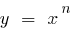where n is any real number, is called power function

## Properties of the power function (in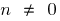)

a) n — natural
b) n is an integer WMAc) n is not a whole

1. Scope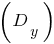2. a)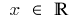b)c) when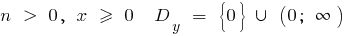when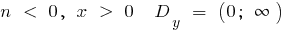3. Multiple value4. a) when n doubles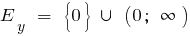when n is odd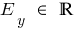b) when n doubles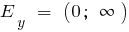when n is odd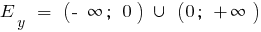c) when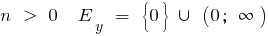when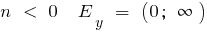5. Parity, odd parity
6. a),b) when n doubles — doubleswhen n is odd — odd

c) neither even nor odd

7. The frequency
8. not periodic

9. The intersection with the coordinate axes
10. a)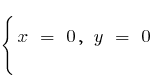b) no

c) when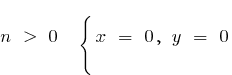when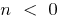— no

11. Derivative
12.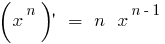13. The increase and decrease of
14. a) when n doubles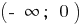— decreases,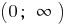increaseswhen n odd — is growing

b) when n doubles— increases— decreaseswhen n odd— comes— comes

c) when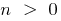— growingwhen itcomes

15. Extrema
16. a) when n doubles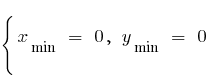when n is odd — no

b) no

c) when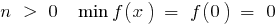when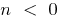— no

17. Asymptotes
18. a) no

b)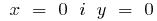c) while— notwhen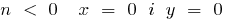19. Convexity and points of inflection
20. a) when n doubles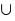— bulge downwhen n is odd,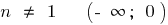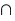;; 0 — inflection point

b) when n doubles —;when n is odd —;c) when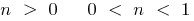;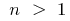when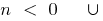21. A special case
22. If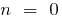,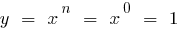(when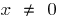)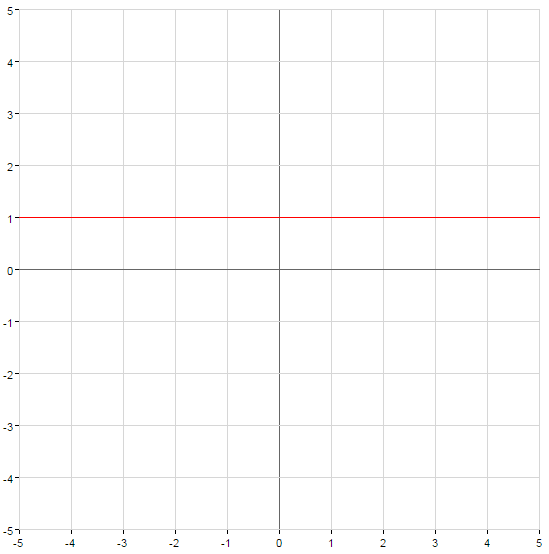## Graphs of power functions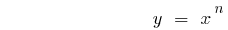• n is an even natural number
•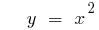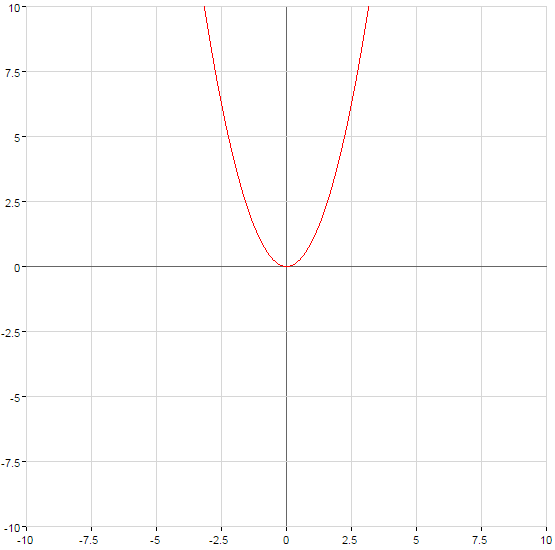• n — an odd natural number
•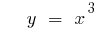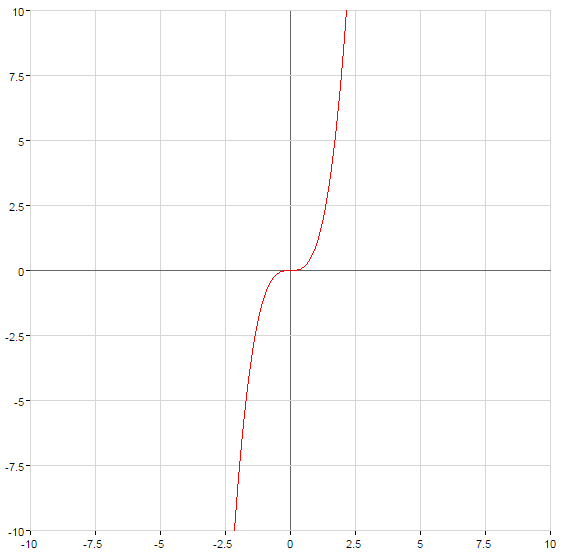• n is an odd number VDA
•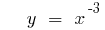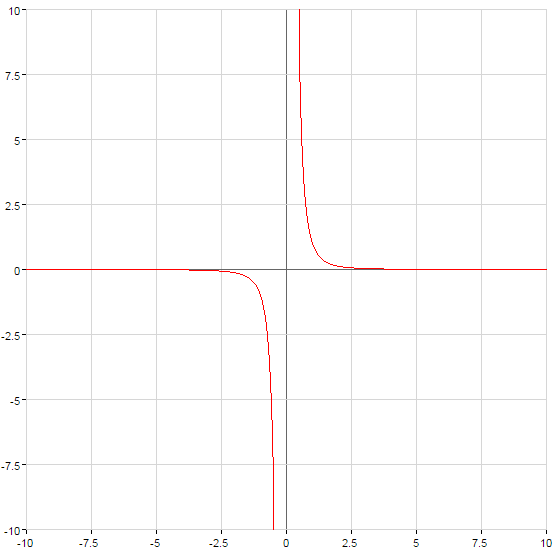• n is an even number VDA
•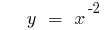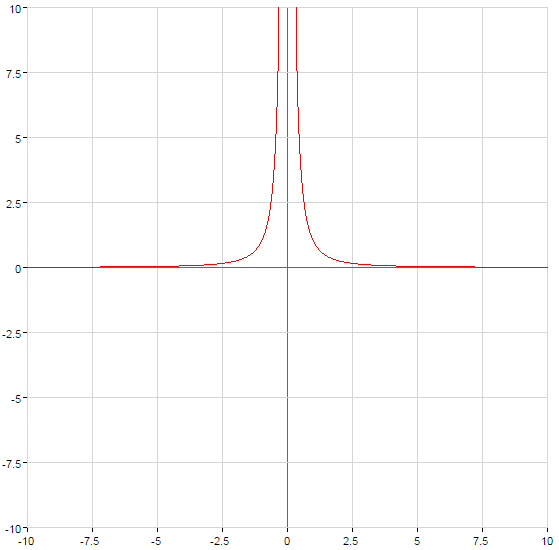• n is not positive integer
•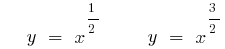(green)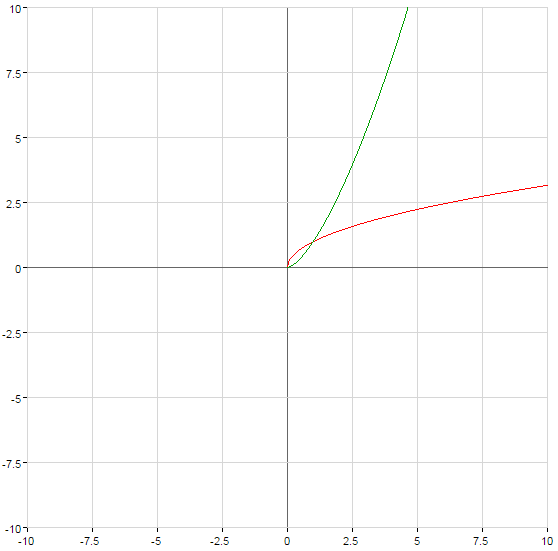• n is not an integer WMA
•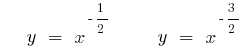(green)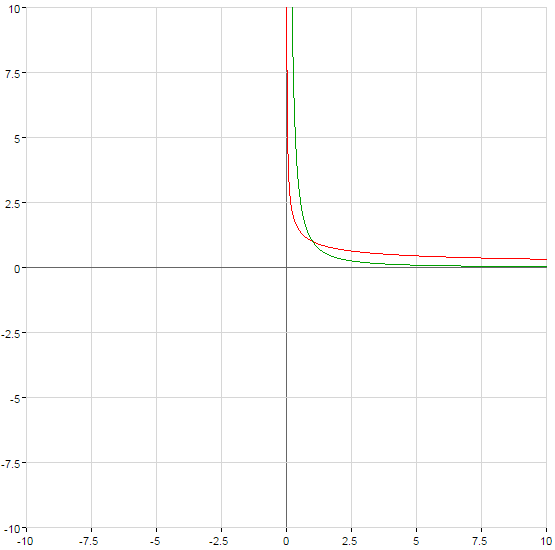Tags:
Chapter:
Versions in other languages:
Share with friends: